# CHSE Odisha Class 12 Math Notes Chapter 5 Determinants

Odisha State Board CHSE Odisha Class 12 Math Notes Chapter 5 Determinants will enable students to study smartly.

## CHSE Odisha 12th Class Math Notes Chapter 5 Determinants

Evaluation of determinants: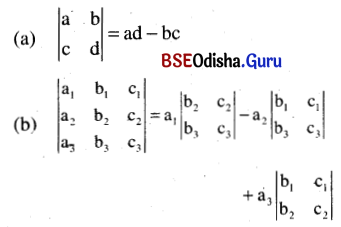Note: We can evaluate a determinant along any row or column.

Minors and cofactors:
Minor of an element aij is the determinant obtained by deleting ith row and jth column denoted by Mij.
Example.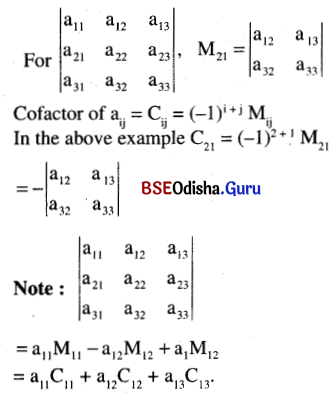Properties of determinant:
(a) The value of a determinant remains unchanged by changing rows to columns and columns to rows.
(b) The interchange of two adjacent rows or columns of determinant changes the sign of a determinant without changing its numerical value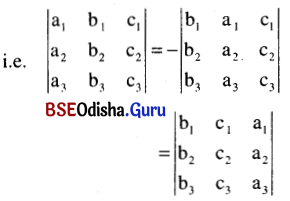(c) If two rows or columns of a determinant are identical then the value of determinant is zero.
(d) If every element of any row or column is multiplied by a factor, then the determinant is multiplied by that factor.
(e) If every element of any row (or column) of a determinant is expressed as sum of two or more numbers, then the determinant can be expressed as the sum of two or more determinants.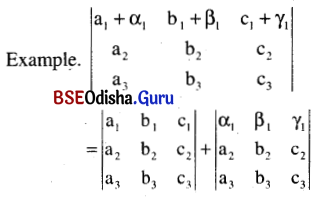(f) A determinant remains unchanged by adding k times the elements of any row (or column) to corresponding elements of any other row (or column) where k is any number or function.

Cramer’s rule to solve a system of linear equations:
Let
a1x + b1y + c1z = d1
a2x + b2y + c2z = d2
a3x + b3y + c3z = d3
is system of linear equations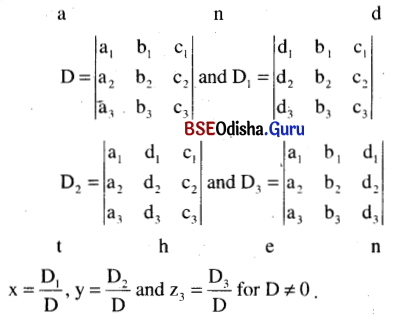Note:
(i) If D1 = D2 = D3 = D = 0 then the system has infinitely many solutions.
(ii) If D = 0 and atleast one of D1, D2 or D3 is not zero then the system has no solution.